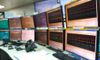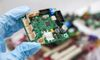# A brief overview of holonomic systems and their usability in robotics10 Feb, 2023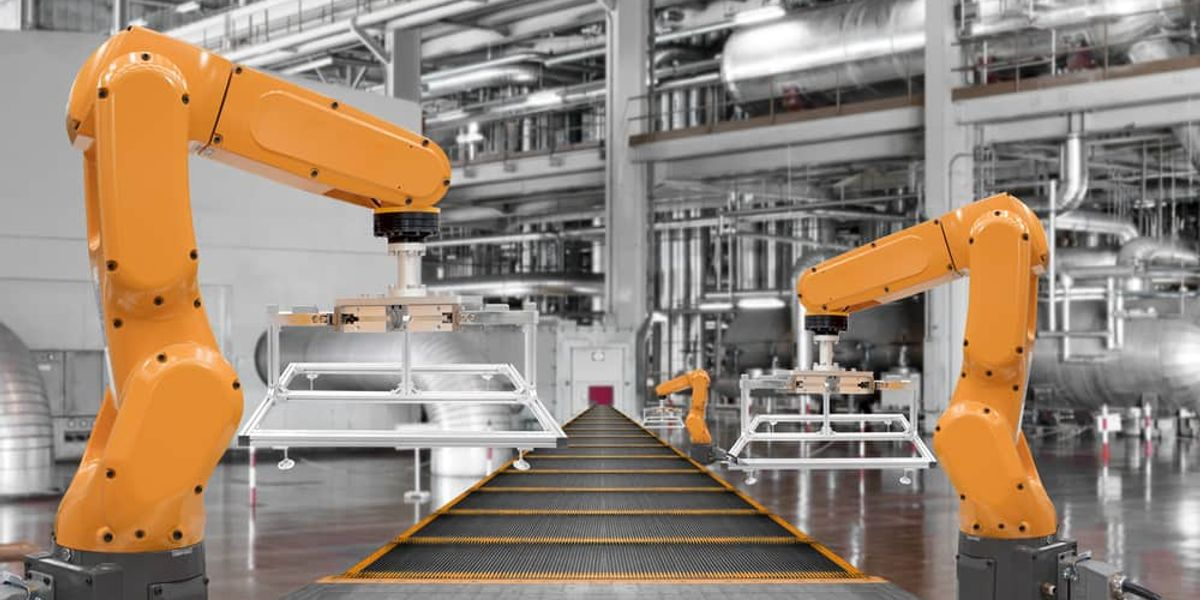holonomic systems and their usability in robotics

## Holonomic systems are important because they allow for efficient mathematical modeling and control of physical systems that have constraints on their motion.

In Newtonian mechanics, the solution to a problem in dynamics was to mark the forces and accelerations on various parts of the system and then apply the equation F=ma in two different directions for a 2-dimensional problem. If a mass or a moment of inertia is not constant, then the equation is F=p. The equation of motion in which the different equations are integrated with respect to space and time to find the desired solution. But sometimes, it is not all that easy to find the equation of motion, as explained above.

An alternative approach to do this is with Lagrangian mechanics, which allows the engineer to find the equation of motion when the Newtonian method is facing difficulties. In Lagrangian mechanics, the engineer has to draw the velocity vectors and then write down the kinetic energy of the system. If the forces are conservative forces, then potential energy also has to be considered. These equations of motion involved kinetic and potential energies.

Holonomic systems are important because they allow for efficient mathematical modeling and control of physical systems that have constraints on their motion. These physical systems have some properties, such as integrability and conservation laws, that make them easier to analyze and predict than non-holonomic systems. These constraints can be seen in various physical systems, such as robots, vehicles, and mechanical systems, and the use of holonomic constraints can lead to more accurate and efficient control algorithms for these systems.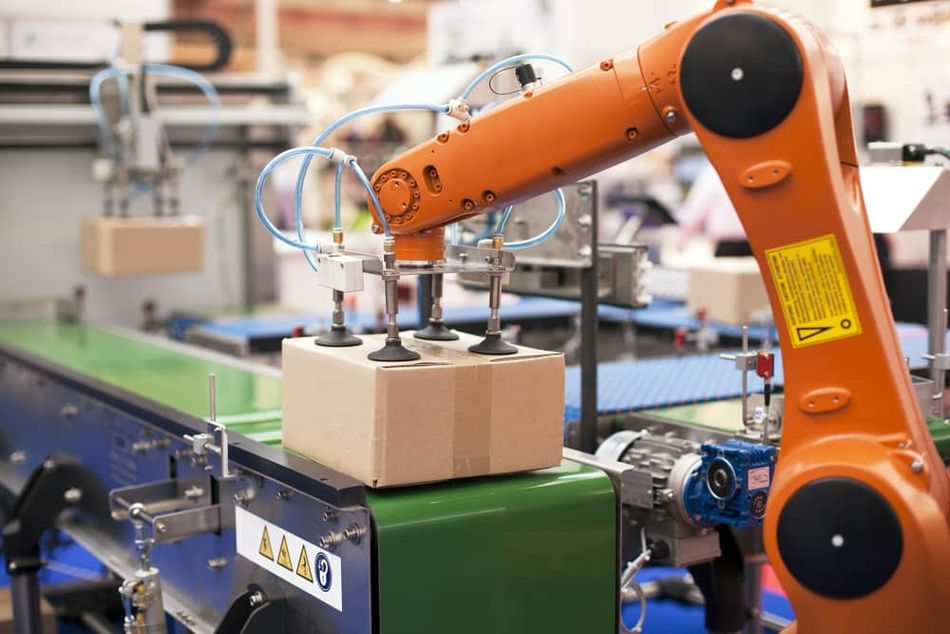Holonomic systems in robotic applications

## What is a holonomic system?

A holonomic system is considered a type of physical system in which the state of the system can be understood by a set of independent and globally defined variables, which are also known as generalized coordinates. These coordinates are used to describe the position and orientation of the system in space. They are sufficient to determine the state of the system. The number of coordinates in such a system is equal to the number of degrees of freedom of the system. They are better understood with the help of holonomic constraints, which are conditions that must be true by the coordinates and the derivatives.

The number of degrees of freedom in a system is important to know the number of independent ways the particle can move. A free particle in a 3-dimensional space has three degrees of freedom. If there are N free particles, there are 3N degrees of freedom. But if there are constraints, the number of degrees of freedom can be reduced. This can be understood by explaining a particle in 3D space with constraint conditions to the surface of a sphere. It can only move in two dimensions, then the degree of freedom is two. To calculate the number of degrees of freedom, the user must know how many independent directions each particle can move in after applying the constraint conditions.

It is also not easy to choose generalized coordinates, and there’s no perfect way to find the best set of coordinates. As in many situations, polar coordinates are considered to be good for circular symmetry in the constraints, spherical coordinates are good for spherical symmetry, and cylindrical coordinates are often good when there is cylindrical symmetry. The whole purpose of generalized coordinates is that they can be efficiently used to handle a problem and take advantage of the fact that Lagrange’s equations are valid, as well as for Cartesian coordinates.

A constraint that can be described by an equation relating to the coordinates is called a holonomic constraint, and the equation that describes the constraint is holonomic. As mentioned earlier, if a system with N particles is subject to k holonomic constraints, the point in 3N-dimensional space that describes the system at any time is not free to move anywhere in 3N-dimensional space but is constrained to move over a surface of dimension 3N-k.

Understanding this with an example from the book “Classical Mechanics,” by Jeremy Tatum of the University of Victoria. Consider a double pendulum, as shown in the figure, and support that the pendulum is constrained to swing only in the plane of the paper or of the screen of your computer monitor. The two unconstrained particles would require six coordinates to specify their positions, but this system is subject to four holonomic constraints. The holonomic equations z1=0 and z2=0 constrain the particles to be moving in a plane, and if the strings are kept taut, we need to have additional holonomic constraints of x1^2 + y1^2=l1^2 and (x2-x1)^2 + (y2-y1)^2= l2^2. Thus, only two coordinates are needed to describe the system, and they could conveniently be the angles the two strings make with the vertical.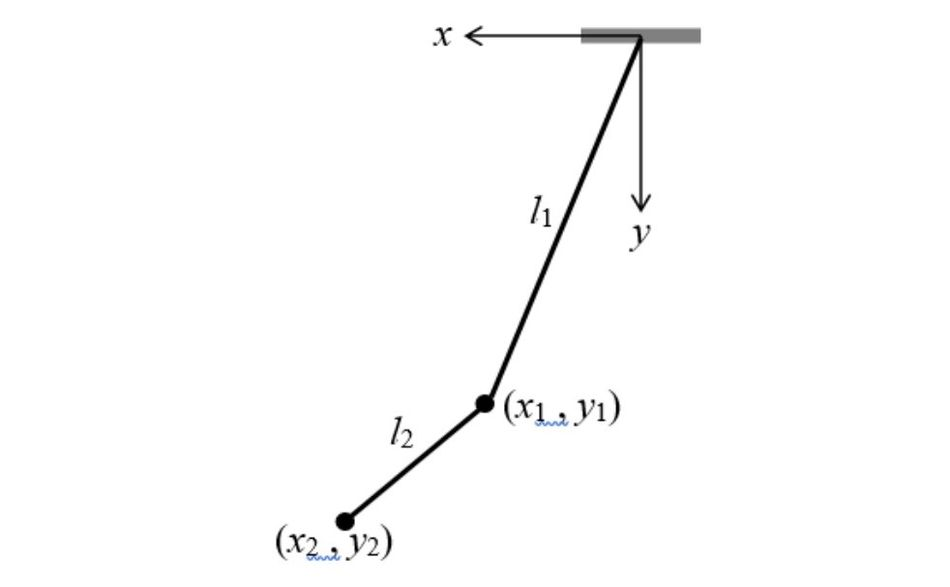Double pendulum is constrained to swing only in the plane of the paper – or of the screen of your computer monitor

## Where are holonomic systems used?

Holonomic constraints are also applied in autonomous vehicles to ensure that the vehicle moves safely and efficiently. They are typically used in the design and control of vehicles, and the constraints on the movement of a vehicle are related to its velocity and position. They are implemented using control algorithms, which take into consideration the vehicle’s velocity, position, and surrounding environment, and use this information to generate control inputs that must satisfy the holonomic constraints. For example, a self-driving car must maintain a minimum safe distance from other vehicles on the road.

Holonomic constraints are widely used in robotic development to model the movement of robots in a mathematically predictable approach. Robots usually have specific degrees of freedom and constraints on their movement, and modeling this using holonomic constraints can simplify the mathematical representation of the robot’s motion. This allows for a more accurate prediction of the robot’s behavior. For example, modeling a wheeled robot with holonomic constraints can simplify the calculation of its motion and make it easier to control its movement. Also, holonomic constraints can ensure that the robot moves safely and stably, as they can be used to enforce constraints such as avoiding collision and staying within a certain workspace.

Recommended reading: Robotic Joint: How Robotic Solution is Boosting Efficiency

## Holonomic constraints in robotics

Constraints are relations between the coordinates due to the physical situation, also known as auxiliary conditions. If the system is constrained, it cannot do something that it could if it didn’t have the restriction placed upon it. For example, if two people are in a room with an open door, they can move in and out of the room. But if the door is locked, then neither of the people can leave the room– this is where they are constrained to stay inside, and the constraint on the system dictates their motion in the locked door.

The constraint system’s equations of motion must satisfy the restriction. For example, any equation of motion about the people locked inside the closed door must exclude solutions where they are outside. A constraint is the rigid body constraint, which for our example, can be expressed as approximating a fixed bond length when calculating rotations, but there are many more. The different constraints can be holonomic and non-holonomic, but the most general constraints can be written as a function of position, velocity, and time. If the constraint is dependent only on the position and not the differentials, then this is the case of holonomic constraints. But if the constraints are a set of constant values, it is an isoperimetric constraint.

Modeling robots with holonomic constraints involves representing the restrictions on their movement as mathematical equations or algorithms. The goal is to express the constraints in a way that can be used to generate control inputs that satisfy the constraints. There are several methods for modeling holonomic constraints, including:

1. Lagrange formulation: This method involves representing the constraints as equations that describe the relationship between the robot's position and velocity. The equations are then combined with the equations of motion to form a system of differential equations that can be used to generate control inputs.

2. Task space formulation: This method involves modeling the robot's movement in a task space, rather than in its joint space. The task space represents the desired movement of the end-effector of the robot, and the holonomic constraints are expressed as equations that limit the movement in this space.

3. Path planning algorithms: This method involves generating a desired path for the robot to follow, and using algorithms to generate control inputs that satisfy the holonomic constraints and guide the robot along the path.

Once the holonomic constraints have been modeled, control algorithms can be used to generate control inputs that satisfy the constraints. These algorithms may be based on classical control methods, such as proportional-integral-derivative (PID) control, or more advanced methods, such as model predictive control (MPC) or reinforcement learning. Overall, the choice of method for modeling holonomic constraints will depend on the specific requirements of the robot and its environment, as well as the desired level of accuracy and control.

1. Increased flexibility: Holonomic drive systems allow for more versatile and flexible movement compared to non-holonomic systems, as the number of wheels or the orientation of the wheels does not limit them. This makes them well-suited for robots that need to move in various directions, such as mobile robots or service robots.

2. Efficient movement: Holonomic drive systems can move in any direction without having to turn around, which makes them more efficient for navigating complex environments or for covering large distances.

3. Improved stability: Holonomic drive systems have improved stability compared to non-holonomic systems, as they can maintain stability even when their wheels are not aligned with their direction of movement.

1. Complexity: Holonomic drive systems are typically more complex and difficult to control compared to non-holonomic systems, as they require more sophisticated control algorithms and sensors to generate control inputs that satisfy the holonomic constraints.

2. Increased cost: Holonomic drive systems are typically more expensive compared to non-holonomic systems due to the increased complexity and the need for additional components, such as sensors and control algorithms.

3. Reduced speed: Holonomic drive systems may be limited in terms of their maximum speed compared to non-holonomic systems, as they may not be able to generate as much traction or generate as much force as non-holonomic systems.

The choice between a holonomic drive system and a non-holonomic drive system will depend on the specific requirements of the robot and its environment, as well as the desired level of accuracy, flexibility, and stability.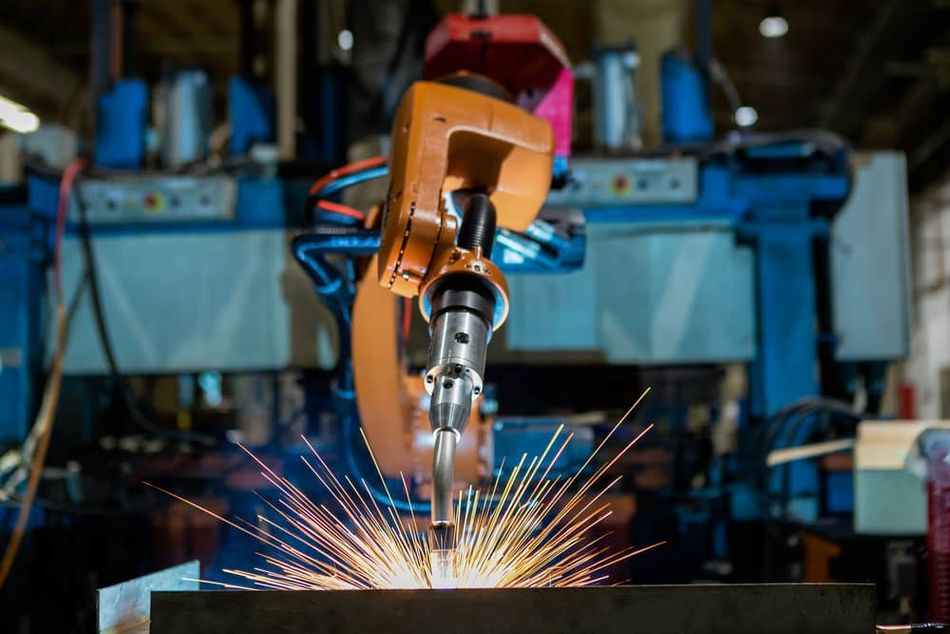Holonomic systems provide increased flexibility, efficient movement, and improved stability

## What is the difference between a Holonomic and a Non-Holonomic Drive?

A holonomic drive system and a non-holonomic drive system are two different types of drive systems used in robots and vehicles. A holonomic drive system refers to a drive system that allows a robot or vehicle to move in any direction, independent of its orientation. This is achieved by having more than two degrees of freedom in the movement of the robot or vehicle. For example, a holonomic drive system for a mobile robot might consist of three or more wheels that can move in any direction, allowing the robot to move in any direction without having to turn around.

A non-holonomic drive system, on the other hand, refers to a drive system that is limited by the number of wheels or the orientation of the wheels. For example, a non-holonomic drive system for a mobile robot might consist of two wheels that can only move in a forward or backward direction. Non-holonomic drive systems are typically simpler and less expensive than holonomic drive systems, but they are also less flexible and less stable, as they are limited in their ability to move in any direction.

The main difference between holonomic and non-holonomic drive systems is the level of freedom in the movement of the robot or vehicle. Holonomic drive systems offer more freedom and flexibility in movement, while non-holonomic drive systems are simpler and less expensive, but offer less freedom and flexibility in movement.

## Key Takeaways

1. An alternative approach to formulating motion equations is with Lagrangian mechanics, which allows the engineer to the equation when the Newtonian method is facing difficulties.

2. A holonomic drive system refers to a drive system that allows a robot or vehicle to move in any direction, independent of its orientation.

3. Holonomic systems are important because they allow for efficient mathematical modeling and control of physical systems that have constraints on their motion.

4. Constraints are relations between the coordinates due to the physical situation, also known as auxiliary conditions.

5. There are several methods for modeling holonomic constraints– Lagrange formulation, task space formulation, and path planning algorithm.

6. Once the holonomic constraints have been modeled, control algorithms can be used to generate control inputs that satisfy the constraints.

7. Advantages of Holonomic drive– Increased flexibility, efficient movement, and improved stability.

8. Disadvantages of Holonomic drive– Complexity, increased cost, and reduced speed.

9. The choice between a holonomic drive system and a non-holonomic drive system will depend on the specific requirements of the robot and its environment, as well as the desired level of accuracy, flexibility, and stability.

10. A difference between holonomic and non-holonomic drive systems is the level of freedom in the movement of the robot or vehicle.

## References

1. Shervani Kh. Soltakhanov , Mikhail P. Yushkov , Sergei A. Zegzhda. Mechanics of non-holonomic systems. 21 October 2010. https://doi.org/10.1007/978-3-540-85847-8

2. Theodore Frankel, University of California, San Diego. Holonomic and Nonholonomic Constraints. June 2012. https://doi.org/10.1017/CBO9781139061377

3. Jeremy Tatum, University of Victoria. Holonomic Constraints. March 5, 2022. https://phys.libretexts.org/Bookshelves/Classical_Mechanics/Classical_Mechanics_(Tatum)/13:_Lagrangian_Mechanics/13.03:_Holonomic_Constraints

4. - Prof Alonzo Kelly, CMU RI. Chapter 4. Dynamics. 4.3 Constrained Kinematics and Dynamics. https://www.cs.cmu.edu/~alonzo/books/dyn2.pdf

5. Alice Quillen. PHY411 Lecture notes on Constraints. January 4, 2019. http://astro.pas.rochester.edu/~aquillen/phy411/lecture12.pdf